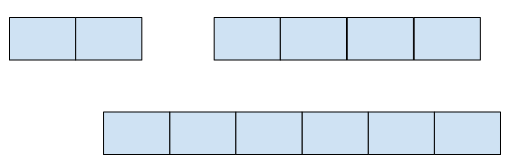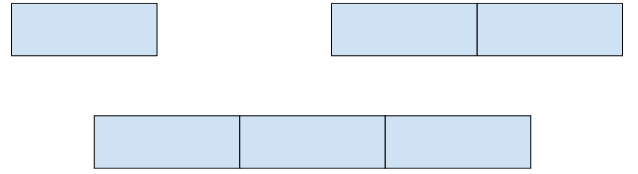# REVSPLIT - Editorial

Author: Abhishek Ghosh
Editorialist: Arkita Aggarwal, Shruti Arya

Simple

# PREREQUISITES

Basic observation, GCD

# PROBLEM

Given an array A consisting of N elements, you need to find the largest number that divides all of them. Minimum outstanding shares will be the sum of this array divided by this maximal number.

# QUICK EXPLANATION

• The highest common factor of the values is found and that is the amount to which share prices can be safely increased.
• The number of shares is then divided by the highest common factor and new number of shares owned is found. The sum of the new values found is the value of minimum outstanding shares.

# EXPLANATION

Each value of the array is taken and divided by incrementing integers one by one till they reach a minimum value. The highest integer will be the greatest common divisor(GCD). This GCD will be the new stock price to which the share price can be increased safely.

All the values of the array are divided by this GCD and those values will be the new number of stocks each investor now owns.
The new values of this array are added to find the minimum outstanding shares.

Example

Consider the three values in the array as 2, 4 \;and \;6In this case the total number of boxes is equal to 12 \;(2+4+6)
The greatest common divisor will be 2 so we can merge two boxes to be considered as 1. This merging of 2 boxes into 1, represents the increase in stock price, and consolidation of shares.Now, the total number of boxes is equal to 6 \;(1+2+3)

# TIME COMPLEXITY

GCD of 2 numbers is computed in O(log(max(A_{i}))) time. This is done for N elements of the array, so the complexity is O(N \cdot log(max(A_{i})).

# SOLUTIONS

Setter's Solution
#include <bits/stdc++.h>
using namespace std;
typedef long long ll;

int32_t main() {
#ifndef ONLINE_JUDGE
freopen("input.txt", "r", stdin);
freopen("output.txt", "w", stdout);
#endif
ios_base::sync_with_stdio(false);
cin.tie(NULL);
cout.tie(NULL);

int T = 1;
cin >> T;

while(T--) {
int n; cin >> n;
vector<int> arr(n);
for(int &x : arr)
cin >> x;
int g = arr;
for(int i = 1; i < n; i++) {
g = __gcd(g, arr[i]);
}
ll sum = 0;
for(int x : arr) {
sum += x/g;
}
cout << sum << "\n";
}

return 0;
}